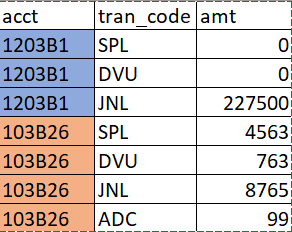# Exclude an ID if its value is non-zero for only one specific category and zero for the rest of them else include itAs shown in the image, I want to exclude the acct if (amt <> 0 for tran_code=JNL and amt = 0 for rest of the tran_code). In any other case, include this account.

I am having trouble achieving this. Can someone please guide me here?

declare @t table (acct varchar(10), tran_code char(3), Amount int)

insert into @t
values
('1203B1','SPL',0),
('1203B1','DVU',0),
('1203B1','JNL',227500),
('103B26','SPL',4563),
('103B26','DVU',763),
('103B26','JNL',7665),

;with cte as
(select Acct,
sum(Case when tran_code = 'JNL' and Amount <> 0 then 1 else 0 end) as JNLNot0,
sum(Case when tran_code <> 'JNL' and Amount = 0 then 1 else 0 end) as Amount0,
count(1) as TotalRecords
from @t
group by acct)

select t.*
from @t t
join cte c
on t.acct = c.acct
and c.JNLNot0 + c.Amount0 <> c.TotalRecords

``````;WITH cte_acct_selection AS (
SELECT acct
FROM @t
GROUP BY acct
HAVING SUM(CASE WHEN tran_code = 'JNL' AND Amount <> 0 THEN 1 ELSE 0 END) = 1 AND
SUM(CASE WHEN tran_code <> 'JNL' AND Amount <> 0 THEN 1 ELSE 0 END) = 0
)
SELECT t.*
FROM @t t
INNER JOIN cte_acct_selection cas ON cas.acct = t.acct
ORDER BY t.acct, t.tran_code``````
1 Like